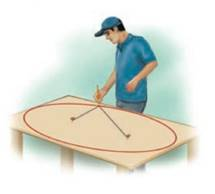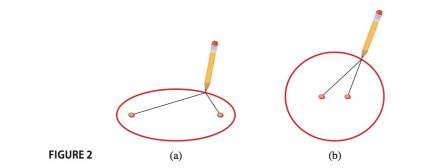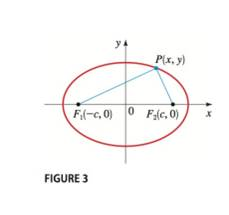Chapter 12.2, Problem 68E### Algebra and Trigonometry (MindTap ...

4th Edition
James Stewart + 2 others
ISBN: 9781305071742

#### Solutions

Chapter
Section### Algebra and Trigonometry (MindTap ...

4th Edition
James Stewart + 2 others
ISBN: 9781305071742
Textbook Problem

# Plywood Ellipse A carpenter wishes to construct an elliptical table top from a 4 ft by 8 ft sheet of plywood. He will trace out the ellipse using the “thumbtack and string” method illustrated in Figure 2 and 3 . What length of string should he use, and how far apart should the tacks be located, if the ellipse is to be the largest possible that can be cut out of the plywood sheet?To determine

To Find:

The length of a string to be used to cut out the plywood sheet and how far apart should the tacks be located.

Explanation

Given:

The length of the major axis is 8ft and length of the minor axis is 4ft.

Approach:

The equation of an ellipse is,

x2a2+y2b2=1

The length of the major axis is 2a.

The length of the minor axis is 2b… (2)

The vertices are (±a,0).

The foci are (±c,0).

Calculation:

From equation (2),

2a=8a=4

and

2b=4b=2

The vertices will be (±4,0)

### Still sussing out bartleby?

Check out a sample textbook solution.

See a sample solution

#### The Solution to Your Study Problems

Bartleby provides explanations to thousands of textbook problems written by our experts, many with advanced degrees!

Get Started